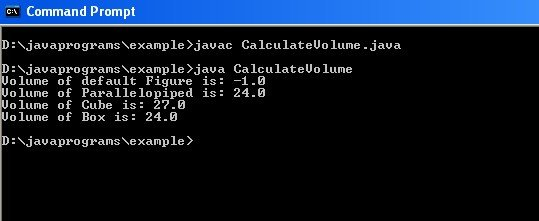We have already discussed all about Java constructors in our previous tutorial and we have also discussed different types of constructors viz. Default Constructor, Parameterized Constructor and Copy Constructor. Before we delve into the details of constructor overloading we will recapitulate constructor once again.

Recapitulate Constructor

• Constructors are used to assign initial values to instance variables of the class immediately upon object creation.
• Constructor is always called by new operator.
• A default constructor with no arguments will be called automatically by the Java Virtual Machine (JVM).
• Constructors are declared just like as we declare methods, except that the constructor doesn’t have any return type.

When the constructors have same name with different arguments (may differ in number/type/sequence of arguments), so called Constructor Overloading.

#### Properties:

• In a class, the concept of constructor overloading does not allow the external user to be aware about the internal processing of the system.
• Overloaded constructors differ in either number of parameters or type of parameters or sequence of parameters.
• JVM differentiates constructors on the basis following criteria
1. Number of Parameters.
2. Type of Parameters.
3. Sequence of Parameters.
• Constructor overloading, allows the user to achieve the compile time polymorphism.

## Program

```class Figure{
double width;
double height;
double depth;
/* Default Constructor */
Figure(){
width = -1;
height = -1;
depth = -1;
}
/* Parameterized constructor when all the dimensions are specified */
Figure(double width,double height,double depth){
this.width = width;
this.height = height;
this.depth = depth;
}
/* Parameterized constructor to calculate volume of Cube */
Figure(double length){
width = height = depth = length;
}
/*Copy Constructor */
Figure(Figure fig){
width = fig.width;
height = fig.height;
depth = fig.depth;
}
/* Method to calculate Volume */
double volume(){
return width*height*depth;
}
}
class CalculateVolume{
public static void main(String args[]){
Figure fig = new Figure();
Figure pp = new Figure(2,3,4);
Figure cube = new Figure(3);
Figure box = new Figure(pp);
System.out.println("Volume of default Figure is: "+fig.volume());
System.out.println("Volume of Parallelopiped is: "+pp.volume());
System.out.println("Volume of Cube is: "+cube.volume());
System.out.println("Volume of Box is: "+box.volume());
}
}```

## Output## Explanation of the Java Code & Output

Here in class Figure, constructor Figure() are overloaded with different arguments in order to initialize instance variables to calculate volume of those geometrical figures. In class Figure we have defines one default constructor to initialize all the instance variables with some default values. Here it is -1. Next two constructors are parameterized constructors where in the first constructor all the dimensions are specified and in the second one dimension for cube is specified. Last one is the copy constructor whenever we need any figure which may be the as same as any of the existing figure. Then we can use this constructor to initialize the dimensions of the figure we will have to create. Thus during object creation JVM differentiates all these overloaded constructors on the basis of resolution criteria mentioned above in the properties.

One more fundamental I would like to recapitulate is being declared parameterized constructor and without declaring default explicitly if you want to create object of that class with default constructor  of that class you will get an error as when you declare parameterized constructor at that time JVM doesn’t provide default constructor from its own. You have to explicitly provide the default constructor while providing parameterized constructor in order to avoid any instantiation error.

N.B: Automatic data type conversion is true for constructor overloading also. This conversion is true for lower datatype( e.g int) to higher datatype (e.g double) conversion not for the reverse (double to int).

Next we will learn about how to return objects from Java methods.

Checkout more useful tutorials and definitive guidelines on Java programming here.

1.Priya says:
2.Cad2print says: